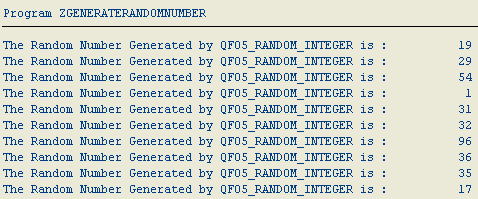﻿ ABAP Tutorial - How to Generate Random Number for a Given Range of Numbers using QF05_RANDOM_INTEGERSAP ABAP Programming and HANA Database Tutorials Development resources, articles, tutorials, code samples, tools and downloads for SAP HANA and ABAP, HANA Database, SQLScript, SAP UI5, Screen Personas, Web Dynpro, Workflow

# ABAP Tutorial - How to Generate Random Number for a Given Range of Numbers using QF05_RANDOM_INTEGER

ABAP developers can use the function module QF05_RANDOM_INTEGER to generate random number for a given range of numbers.
There are several other methods for ABAP random number generation.

Frequently, ABAP developers require to create random integer numbers.
There are a few function modules that can help you generate random numbers in your SAP system.
I want to show the ABAP code for generating random numbers using function module QF05_RANDOM_INTEGER in this ABAP tutorial.
Below you can find the ABAP example and the source codes for ABAP random numbers.

The local variables with integer data type lv_MinI and lv_MaxI are the input parameters.
They define the range of numbers by identifying the start and the end of the numbers range.
lv_RndI is used to get the output generated random number from the QF05_RANDOM_INTEGER function module.

And the output of this example ABAP program that generate random numbers is shown in the below screenshot.
This small ABAP code can be used for random number generation in ABAP.SAP HANA and ABAP